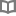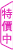寂寞很簡單 - 恩佐 - BOOKWALKER中文電子書

寂寞很簡單

恩佐

恩佐是筆名。取自天使的英文諧音，也是感謝生命中幫助過自己的人。高中時想當漫畫家，後來幾乎停止畫畫；大學考上了新聞系，可是從未考慮當記者；畢業之後跑去書店工作，同時創作投稿。現在是全職作家。學生時代得過幾張獎狀，可是沒有受過正統的美術訓練。對於插畫家的身分，還是不太習慣，最喜歡的還是自己寫自己畫。作品屢獲好評，曾獲得誠品、金石堂最佳圖文書，以及金鼎獎年度最佳圖畫書。目前在雜誌與報紙都有專欄連載，也為多本暢銷書繪製封面插圖。圖文作品海豚愛上熱咖啡（大田）因為心在左邊（大田）最遠的你最近的我（大田）一年甲班34ㄏㄠˋ（時報）幸福練習簿（大田）

• 放入購物車
•試閱• 放入購物車
•試閱

• 放入購物車
•試閱

• 放入購物車
•試閱

• 放入購物車
•試閱

• 放入購物車
•試閱

• 放入購物車
•試閱

• 放入購物車
•試閱• 放入購物車
•試閱

• 放入購物車
•試閱

• 放入購物車
•試閱

• 放入購物車
•試閱

• 放入購物車
•試閱

• 放入購物車
•試閱

• 放入購物車
•試閱

• 放入購物車
•試閱

• 放入購物車
•試閱

• 放入購物車
•試閱

• 放入購物車
•試閱• 放入購物車
•試閱

• 放入購物車
•試閱• 放入購物車
•試閱

• 放入購物車
•試閱• 放入購物車
•試閱

• 放入購物車
•試閱

• 放入購物車
•試閱

• 放入購物車
•試閱

• 放入購物車
•試閱

• 放入購物車
•試閱

• 放入購物車
•試閱

• 放入購物車
•試閱• 放入購物車
•試閱

• 放入購物車
•試閱

• 放入購物車
•試閱• 放入購物車
•試閱

• 放入購物車
•試閱

• 放入購物車
•試閱

• 放入購物車
•試閱• 放入購物車
•試閱

• 放入購物車
•試閱

• 放入購物車
•試閱

• 放入購物車
•試閱

• 放入購物車
•試閱

• 放入購物車
•試閱

• 放入購物車
•試閱

• 放入購物車
•試閱